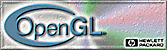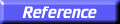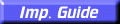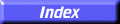# glBlendFunc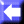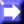glBlendFunc: specify pixel arithmetic.

C Specification | Parameters | Description | Examples | Notes | Errors | Associated Gets | See Also

##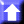C Specification

```void glBlendFunc(
GLenum	 sfactor,
GLenum	 dfactor)
```

##Parameters

sfactor
Specifies how the red, green, blue, and alpha source blending factors are computed. Nine symbolic constants are accepted: GL_ZERO, GL_ONE, GL_DST_COLOR, GL_ONE_MINUS_DST_COLOR, GL_SRC_ALPHA, GL_ONE_MINUS_SRC_ALPHA, GL_DST_ALPHA, GL_ONE_MINUS_DST_ALPHA, and GL_SRC_ALPHA_SATURATE. The initial value is GL_ONE.
dfactor
Specifies how the red, green, blue, and alpha destination blending factors are computed. Eight symbolic constants are accepted: GL_ZERO, GL_ONE, GL_SRC_COLOR, GL_ONE_MINUS_SRC_COLOR, GL_SRC_ALPHA, GL_ONE_MINUS_SRC_ALPHA, GL_DST_ALPHA, and GL_ONE_MINUS_DST_ALPHA. The initial value is GL_ZERO.

##Description

In RGBA mode, pixels can be drawn using a function that blends the incoming (source) RGBA values with the RGBA values that are already in the frame buffer (the destination values). Blending is initially disabled. Use glEnable and glDisable with argument GL_BLEND to enable and disable blending.

glBlendFunc defines the operation of blending when it is enabled. sfactor specifies which of nine methods is used to scale the source color components. dfactor specifies which of eight methods is used to scale the destination color components. The eleven possible methods are described in the following table. Each method defines four scale factors, one each for red, green, blue, and alpha.

In the table and in subsequent equations, source and destination color components are referred to as ( Rs , Gs , Bs , As ) and ( Rd , Gd , Bd , Ad ). They are understood to have integer values between 0 and ( kR , kG , kB , kA ), where

kc = 2mc
1

and ( mR , mG , mB , mA ) is the number of red, green, blue, and alpha bitplanes.

Source and destination scale factors are referred to as ( sR , sG , sB , sA ) and ( dR , dG , dB , dA ). The scale factors described in the table, denoted ( fR , fG , fB , fA ), represent either source or destination factors. All scale factors have range [0,1].

Parameter ( fR, fG, fB, fA )
GL_ZERO (0, 0, 0, 0)
GL_ONE (1, 1, 1, 1)
GL_SRC_COLOR (Rs/kR, Gs/kG, Bs/kB, As/kA)
GL_ONE_MINUS_SRC_COLOR (1, 1, 1, 1)(Rs/kR, Gs/kG, Bs/kB, As/kA )
GL_ONE_MINUS_DST_COLOR (1, 1, 1, 1)(Rd/kR, Gd/kG, Bd/kB, Ad/kA )
GL_SRC_ALPHA (As/kA, As/kA, As/kA, As/kA)
GL_ONE_MINUS_SRC_ALPHA (1, 1, 1, 1)(As/kA, As/kA, As/kA, As/kA )
GL_ONE_MINUS_DST_ALPHA (1, 1, 1, 1)(Ad/kA, Ad/kA, Ad/kA, Ad/kA )
GL_SRC_ALPHA_SATURATE (i, i, i, 1)

In the table,

i = min(As, kAAd) / kA

To determine the blended RGBA values of a pixel when drawing in RGBA mode, the system uses the following equations:

Rd = min(kR, Rs sR + Rd dR)
Gd = min(kG, Gs sG + Gd dG)
Bd = min(kB, Bs sB + Bd dB)

Despite the apparent precision of the above equations, blending arithmetic is not exactly specified, because blending operates with imprecise integer color values. However, a blend factor that should be equal to 1 is guaranteed not to modify its multiplicand, and a blend factor equal to 0 reduces its multiplicand to 0. For example, when sfactor is GL_SRC_ALPHA, dfactor is GL_ONE_MINUS_SRC_ALPHA, and As is equal to kA, the equations reduce to simple replacement:

Rd = Rs
Gd = Gs
Bd = Bs

##Examples

Transparency is best implemented using blend function (GL_SRC_ALPHA, GL_ONE_MINUS_SRC_ALPHA) with primitives sorted from farthest to nearest. Note that this transparency calculation does not require the presence of alpha bitplanes in the frame buffer.

Blend function (GL_SRC_ALPHA, GL_ONE_MINUS_SRC_ALPHA) is also useful for rendering antialiased points and lines in arbitrary order.

Polygon antialiasing is optimized using blend function (GL_SRC_ALPHA_SATURATE, GL_ONE) with polygons sorted from nearest to farthest. (See the glEnable, glDisable reference page and the GL_POLYGON_SMOOTH argument for information on polygon antialiasing.) Destination alpha bitplanes, which must be present for this blend function to operate correctly, store the accumulated coverage.

##Notes

Incoming (source) alpha is correctly thought of as a material opacity, ranging from 1.0 (KA), representing complete opacity, to 0.0 (0), representing complete transparency.

When more than one color buffer is enabled for drawing, the GL performs blending separately for each enabled buffer, using the contents of that buffer for destination color. (See glDrawBuffer.)

Blending affects only RGBA rendering. It is ignored by color index renderers.

##Errors

• GL_INVALID_ENUM is generated if either sfactor or dfactor is not an accepted value.
• GL_INVALID_OPERATION is generated if glBlendFunc is executed between the execution of glBegin and the corresponding execution of glEnd.

##Associated Gets

glGet with argument GL_BLEND_SRC
glGet with argument GL_BLEND_DST
glIsEnabled with argument GL_BLEND

glAlphaFunc,
glClear,
glDrawBuffer,
glEnable,
glLogicOp,
glStencilFunc